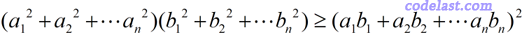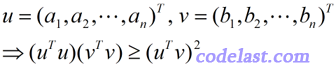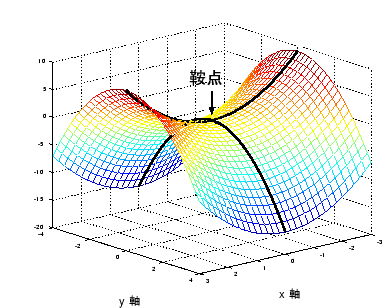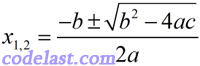# Math concepts / 数学概念

Following are some basic math concepts I read or summarized in my learning process, I wrote them down here to share with those who need them.

（1）奇异函数

（2）奇点

“奇点”是复变函数里的一个概念。在一个区域内可导的复变函数，称为这个区域内的解析函数，如果一个复变函数在挖掉点z的区域内解析，但在点z处不解析，则z称为这个解析函数的奇点。解析函数的奇点总是孤立的，奇点按其性质，可以分为：可去奇点、极点和本性奇点三大类。

（3）病态多项式

“病态多项式”是与病态代数方程的概念相关的。

$\begin{array}{l}p(x) = (x - 1)(x - 2)(x - 3) \cdots (x - 7)\\= {x^7} - 28{x^6} + 322{x^5} - 1960{x^4} + 6769{x^3} - 13132{x^2} + 13068x - 5040\end{array}$

（4）超线性收敛

A）线性收敛： $\frac{{\left\| {{x^{\left( {k + 1} \right)}} - {x^ * }} \right\|}}{{\left\| {{x^{\left( k \right)}} - {x^ * }} \right\|}} < 1$  ，当 $k$ 充分大时成立

B）超线性收敛： $\mathop {\lim }\limits_{k \to \infty } \frac{{\left\| {{x^{\left( {k + 1} \right)}} - {x^ * }} \right\|}}{{\left\| {{x^{\left( k \right)}} - {x^ * }} \right\|}} = 0$

C）二阶收敛： $\exists \alpha > 0$  ，当 $k$ 充分大时有：  $\frac{{\left\| {{x^{\left( {k + 1} \right)}} - {x^ * }} \right\|}}{{{{\left\| {{x^{\left( k \right)}} - {x^ * }} \right\|}^2}}} \le \alpha$

（6）最小二乘的理论依据
http://www.codelast.com/?p=1027

（7）Powell算法
http://www.codelast.com/?p=388

（8）黄金比例搜索算法
http://www.codelast.com/?p=434

（9）奇异方程组

（10）奇异值分解

（11）主元

（12）完全主元法 & 部分主元法

（13）矩阵的初等变换
a、交换矩阵的两行（列）；

b、用一个不为零的数乘矩阵的某一行(列)；

c、用一个数乘矩阵某一行（列）加到另一行（列）上。

（14）外推法

（15）Ridders求导算法
http://www.codelast.com/?p=1419

（16）线性/非线性规划

（17）凸集

（18）半正定矩阵
n×n的矩阵M，若对于任意非零的x∈Rn，有xTMx≥0，则称M为半正定矩阵。

（19）奇异矩阵

（21）水平集

（22）由两两线性无关的列向量构成的矩阵是满秩的

（23）线性流形

“流形”（manifold）是数学中用于描述几何形体的一个概念，它是指局部具有欧几里得空间性质的空间。 欧几里得空间就是最简单的流形的实例。欧几里得空间也被理解为线性流形。

（24）满秩与正定的一个关系

（25）二次型

（26）正定二次型

（27）正定矩阵均可逆，并且其逆也是正定矩阵

（28）柯西不等式/柯西-施瓦茨不等式/Cauchy–Schwarz inequality（29）大O和小o：同阶无穷小与高阶无穷小

（30）稀疏矩阵：元素大部分为0的矩阵

（31）关于正定矩阵共轭的非零向量组线性无关

（32）实对称矩阵A正定的充分必要条件是存在可逆矩阵C，使得 A=CTC。由于可逆矩阵是正定矩阵，所以对称正定矩阵A满足：存在正定矩阵D，使得A=DTD

（33）每一个秩一矩阵都可以化为一个列向量与一个行向量之积

（34）驻点及鞍点（35）雅可比矩阵（Jacobi matrix）不一定是方阵（n×n的矩阵）

（36）无解的线性方程组被称为是不相容的，有一或无穷多个解的线性方程组被称为是相容

（37）若一个矩阵经过一系列行初等变换可以变成另一个矩阵，则称这两个矩阵是行等价

（38）一元二次方程 $a{x^2} + bx + c = 0\;(a \ne 0)$ 的求根公式